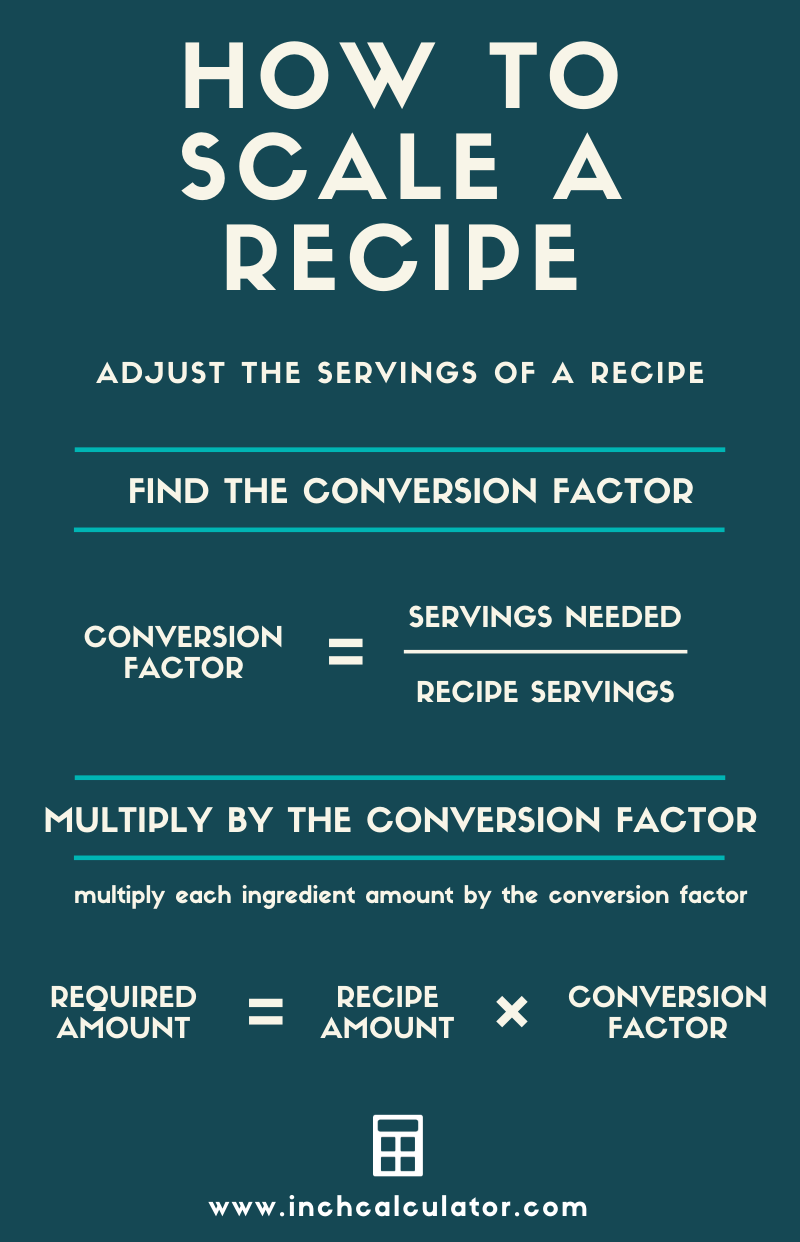# Recipe Scale Conversion Calculator – Recipe Multiplier

If you find the process of scaling a recipe up or down confusing or tedious like we do, then you’re going to love this recipe scaling calculator!

Enter your ingredients, and the calculator will increase or decrease them automatically.

Portions
portions
portions

## Scaled Recipe Ingredients:

Learn how we calculated this below

## How to Scale a Recipe

You can increase or decrease the portions of a recipe in just a few easy steps. Follow along as we show how to scale the yield of a recipe up or down.### Step One: Find the Conversion Factor

The first thing we need to do to adjust recipe quantities is to find the conversion factor that we want to increase or decrease the recipe size.

If you know how many portions the recipe makes, and you know how many portions you want, then use this formula to find the conversion factor.

conversion factor = portions needed ÷ recipe portions

Kitchen professionals and chefs refer to the number of portions as the yield. Thus, the conversion factor is the required yield divided by the recipe’s yield.

So, if you want to double the recipe size, then the factor is 2; if you’re going to cut the recipe in half, then the conversion factor is .5.

Hint: you can use a tool such as our scale calculator to find the scale factor.

### Step Two: Adjust the Recipe Using the Conversion Factor

The next step in scaling a recipe is to adjust the amount of each ingredient using the conversion factor.

To do this, multiply each ingredient portion by the conversion factor to get the adjusted amount. If the conversion factor is two and the recipe calls for three tablespoons of an ingredient, then the scaled recipe would use six tablespoons (2 × 3 = 6).

### Step Three: Convert Measurements

The final step is to convert measures to the appropriate measure. This is an optional step but will simplify the recipe.

When you scale a recipe, the measures used might not be appropriate any longer. If a recipe calls for 4 ounces and the recipe is quadrupled, then the new amount would be 16 ounces. Since there are 16 ounces in a pound, you can convert the ingredient quantity from 16 ounces to 1 pound.

You can use our cooking conversion calculator or the following chart of common kitchen conversions to help convert the ingredient quantities.

Conversions of common kitchen and baking measurements
Measurement Equivalent Measurement
1 tablespoon 3 teaspoons
1 fluid ounce 2 tablespoons
1 cup 8 fluid ounces
1 pint 2 cups
1 quart 2 pints
1 gallon 4 quarts
1 pound 16 ounces

Try our volume and weight conversion calculators to simplify converting from a smaller unit to a larger one

## Things to Consider When Scaling a Recipe

We need to highlight a few things you should consider when scaling a recipe.

### Measuring by Weight vs. Volume

First, when measurements are in volumes, scaling the yield up magnifies the inaccuracy of measuring by volume. Consider converting to weight first. See our sugar and flour conversion calculators to convert from volume to weight.

When appropriate, dry ingredients should be measured by weight rather than volume to improve the accuracy of the recipe. Dry ingredients vary slightly in density and can be compacted differently when measuring.

Most experts agree that dry ingredients should be measured by weight rather than volume. We suggest converting the ingredient to a weight measurement before using the calculator above.

### Cooking and Baking Times May Change

An additional consideration to be aware of when increasing or decreasing the size of a recipe is that mixing and cooking/baking times can vary as the amount of the ingredients changes. For instance, doubling the size of a cake might require it to cook longer, at a different temperature, or both.

## References

1. The BC Cook Articulation Committee, Basic Kitchen and Food Service Management, https://ecampusontario.pressbooks.pub/basickitchenandfoodservicemanagement/chapter/convert-and-adjust-recipes-and-formulas/
2. National Institute of Standards & Technology, Culinary Measurement Tips, https://www.nist.gov/pml/owm/culinary-measurement-tips